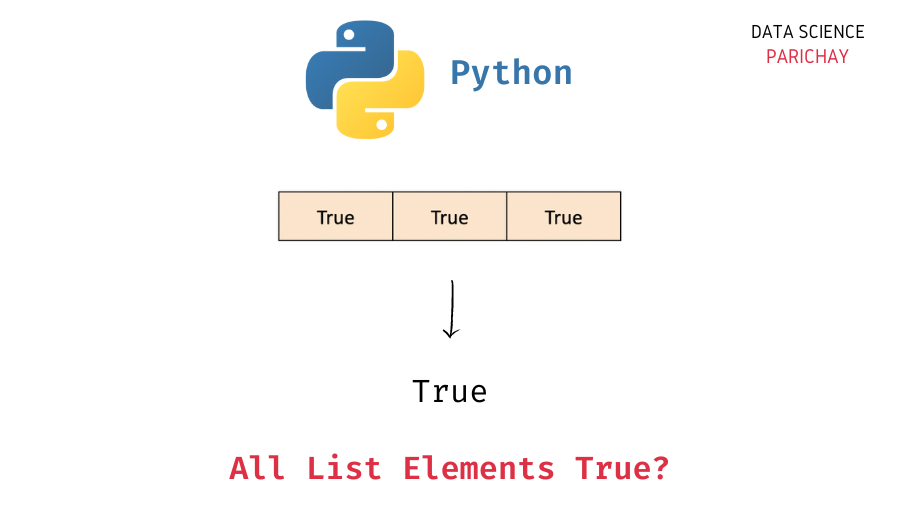# Python – Check if All Elements in List are True

In this tutorial, we will look at how to check if all the elements in a list are True or not in Python with the help of some examples.

## How to check if all the list values are True?

You can use the Python built-in `all()` function to check if all the elements in a list are True or not.

The `all()` function takes in an iterable as an argument and returns `True` if all the values in the iterable are truthy (represent `True` in a boolean context).

Now, if you want to check whether all the values in a list evaluate to True in a boolean context, directly pass the list as an argument to the `all()` function.

```# check if all the list values are truthy
all(ls)```

If, on the other hand, you want to check whether each value in the list is the boolean value `True`, then inside the `all()` function, use an iterator to check whether each list value is `True` or not.

```# check if all the list values are True
all(val == True for val in ls)```

It returns `True` if all the values in the list are True.

## Examples

Let’s now look at some examples of using the above methods. First, we will create a few lists that we’ll use to demonstrate the methods.

```# list with all True values
ls1 = [True, True, True, True]
# list with not all values True
ls2 = [False, True, False, True]
# empty list
ls3 = []

# display the lists
print(ls1)
print(ls2)
print(ls3)```

Output:

📚 Data Science Programs By Skill Level

Introductory

Intermediate ⭐⭐⭐

🔎 Find Data Science Programs 👨‍💻 111,889 already enrolled

Disclaimer: Data Science Parichay is reader supported. When you purchase a course through a link on this site, we may earn a small commission at no additional cost to you. Earned commissions help support this website and its team of writers.

```[True, True, True, True]
[False, True, False, True]
[]```

Here, we created three lists – `ls1`, `ls2`, and `ls3`. The list `ls1` contains only `True` as its elements. The list `ls2` has repeated values but not all values are `True` and the list `ls3` is empty (it does not contain any elements).

### Example 1 – Check if all the list elements are True using `all()`

As mentioned earlier, the `all()` function returns `True` if all the values in the iterable are truthy (evaluate to `True` in a boolean context).

Let’s now apply the `all()` function to the lists created above.

```# check if all values in list evaluate to True
print(all(ls1))
print(all(ls2))
print(all(ls3))```

Output:

```True
False
True```

We get `True` for `ls1` and `False` for `ls2`. Note that if you apply the `all()` function to an empty list, you get `True` as the result.

### Example 2 – Using `all()` on list with non-boolean values

Note that the `all()` does not exactly check for whether the individual values are True or False. Instead, it checks whether the individual values evaluate to `True` or `False` in a boolean context.

For example, the value `1` evaluates to `True` in a boolean context. Similarly, the values `0` and `None`, empty collections (such as lists), etc. evaluate to `False` in a boolean context. Non-empty collections, numeric values other than `0`, etc. evaluate to `True` in a boolean context.

Let’s look at an example.

```# list with truthy values
ls4 = [1, 3, 1, True]
print(all(ls4))```

Output:

`True`

Here, we applied the `all()` function to a list containing truthy values (all the values result to `True` in a boolean context) and thus we get `True` as the output.

If, the above list has any falsy (evaluate to `False` in a boolean context) values, the `all()` function will result in `False`.

```ls4 = [0, 3, 1, True]
print(all(ls4))```

Output:

`False`

We get `False` as the result.

Now, depending on your use case, you might only want to check whether the values in a list are truthy or not. In that case, use the `all()` function directly and pass the list (or the iterable) as an argument.

If, on the other hand, you want to check whether all the values in a list are exactly equal to the boolean value `True`, then, inside the `all()` function, check if each value is exactly equal to `True` or not using an iterator.

Here’s an example.

```# list with truthy values
ls4 = [1, 3, 1, True]
# check if all values are exactly True
print(all(val == True for val in ls4))```

Output:

`False`

We get `False` as the output even if all the values in the above list are truthy.

## Summary

In this tutorial, we looked at how to check if all the values in a list are True or not. The following are the key takeaways –

• Use the Python built-in `all()` function to check if all the elements in a list are truthy or not.
• In case you want to check whether each value is exactly `True` or not, iterate through each value and check if it’s equal to `True` using an iterator inside the `all()` function.

You might also be interested in –

•